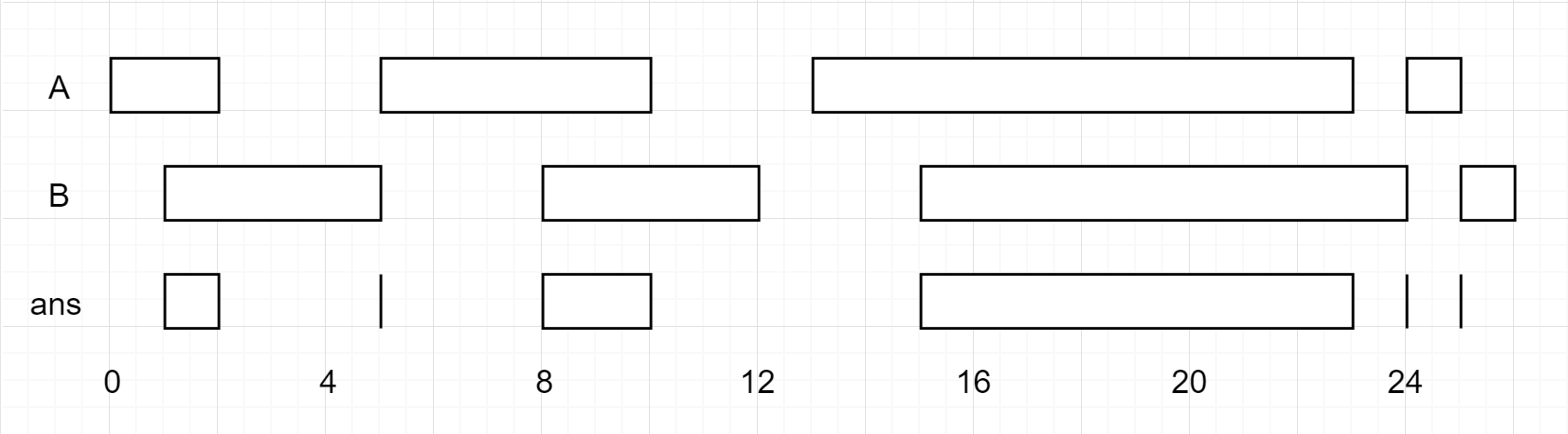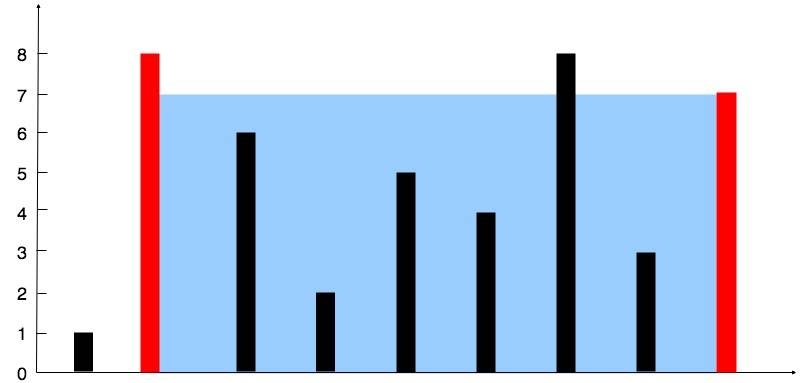# 2022-05-31

## ​844. Backspace String Compare​

### Description

Given two strings `s` and `t`, return `true` if they are equal when both are typed into empty text editors. `'#'` means a backspace character.
Note that after backspacing an empty text, the text will continue empty.
Example 1:
Input: s = "ab#c", t = "ad#c"
Output: true
Explanation: Both s and t become "ac".
Example 2:
Input: s = "ab##", t = "c#d#"
Output: true
Explanation: Both s and t become "".
Example 3:
Input: s = "a#c", t = "b"
Output: false
Explanation: s becomes "c" while t becomes "b".
Constraints:
• `1 <= s.length, t.length <= 200`
• `s` and `t` only contain lowercase letters and `'#'` characters.
Follow up: Can you solve it in `O(n)` time and `O(1)` space?

### Solution

#### Approach #0

func backspaceCompare(s string, t string) bool {
m, n := len(s), len(t)
i, j := m-1, n-1
for i >= 0 || j >= 0 {
i, j = goBack(s, i), goBack(t, j)
if i >= 0 && j >= 0 && s[i] != t[j] {
return false
}
i--
j--
}
return i == j
}
func goBack(s string, i int) int {
count := 0
j := i
for j >= 0 && (s[j] == '#' || count > 0) {
if s[j] != '#' {
count--
} else {
count++
}
j--
}
return j
}

#### Approach #1

func backspaceCompare(s string, t string) bool {
return build(s) == build(t)
}
func build(s string) string {
var st []rune
for _, ch := range s {
if ch == '#' {
n := len(st)
if n > 0 {
st = st[:n-1]
}
} else {
st = append(st, ch)
}
}
return string(st)
}

## ​986. Interval List Intersections​

### Description

You are given two lists of closed intervals, `firstList` and `secondList`, where `firstList[i] = [start[i], end[i]]` and `secondList[j] = [start[j], end[j]]`. Each list of intervals is pairwise disjoint and in sorted order.
Return the intersection of these two interval lists.
A closed interval `[a, b]` (with `a <= b`) denotes the set of real numbers `x` with `a <= x <= b`.
The intersection of two closed intervals is a set of real numbers that are either empty or represented as a closed interval. For example, the intersection of `[1, 3]` and `[2, 4]` is `[2, 3]`.
Example 1:Input: firstList = [[0,2],[5,10],[13,23],[24,25]], secondList = [[1,5],[8,12],[15,24],[25,26]]
Output: [[1,2],[5,5],[8,10],[15,23],[24,24],[25,25]]
Example 2:
Input: firstList = [[1,3],[5,9]], secondList = []
Output: []
Constraints:
• `0 <= firstList.length, secondList.length <= 1000`
• `firstList.length + secondList.length >= 1`
• `0 <= start[i] < end[i] <= 10^9`
• `end[i] < start[i+1]`
• `0 <= start[j] < end[j] <= 10^9`
• `end[j] < start[j+1]`

### Solution

#### Approach #0

func intervalIntersection(firstList [][]int, secondList [][]int) [][]int {
i, j := 0, 0
m, n := len(firstList), len(secondList)
var ans [][]int
for i < m && j < n {
a1, b1 := firstList[i], firstList[i]
a2, b2 := secondList[j], secondList[j]
if a1 <= b2 && b1 >= a2 {
x, y := a1, b2
if a1 <= a2 {
x = a2
}
if b1 <= b2 {
y = b1
}
ans = append(ans, []int{x, y})
}
if b1 < b2 {
i++
} else {
j++
}
}
return ans
}

#### Approach #1

func intervalIntersection(firstList [][]int, secondList [][]int) [][]int {
i, j := 0, 0
var ans [][]int
for i < len(firstList) && j < len(secondList) {
low := max(firstList[i], secondList[j])
high := min(firstList[i], secondList[j])
if low <= high {
ans = append(ans, []int{low, high})
}
if firstList[i] < secondList[j] {
i++
} else {
j++
}
}
return ans
}
func max(a, b int) int {
if a > b {
return a
}
return b
}
func min(a, b int) int {
if a < b {
return a
}
return b
}

## ​11. Container With Most Water​

### Description

You are given an integer array `height` of length `n`. There are `n` vertical lines drawn such that the two endpoints of the `ith` line are `(i, 0)` and `(i, height[i])`.
Find two lines that together with the x-axis form a container, such that the container contains the most water.
Return the maximum amount of water a container can store.
Notice that you may not slant the container.
Example 1:Input: height = [1,8,6,2,5,4,8,3,7]
Output: 49
Explanation: The above vertical lines are represented by array [1,8,6,2,5,4,8,3,7]. In this case, the max area of water (blue section) the container can contain is 49.
Example 2:
Input: height = [1,1]
Output: 1
Constraints:
• `n == height.length`
• `2 <= n <= 10^5`
• `0 <= height[i] <= 10^4`

### Solution

#### Approach #0

func maxArea(height []int) int {
n := len(height)
i, j := 0, n-1
var ans int
for i < j {
ans = max(ans, (j-i)*min(height[i], height[j]))
if height[i] < height[j] {
i++
} else {
j--
}
}
return ans
}
func max(a, b int) int {
if a > b {
return a
}
return b
}
func min(a, b int) int {
if a < b {
return a
}
return b
}